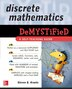# Discrete Mathematics DeMYSTiFied

## bySteven G. Krantz

November 4, 2008|\$34.95
Paperback
Earn 175 plum® points

In stock online

Not available in stores

Prices and offers may vary in store

MULTIPLY your chances of understanding DISCRETE MATHEMATICS

If you're interested in learning the fundamentals of discrete mathematics but can't seem to get your brain to function, then here's your solution. Add this easy-to-follow guide to the equation and calculate how quickly you learn the essential concepts.

Written by award-winning math professor Steven Krantz, Discrete Mathematics Demystified explains this challenging topic in an effective and enlightening way. You will learn about logic, proofs, functions, matrices, sequences, series, and much more. Concise explanations, real-world examples, and worked equations make it easy to understand the material, and end-of-chapter exercises and a final exam help reinforce learning.

This fast and easy guide offers:

• Numerous figures to illustrate key concepts
• Sample problems with worked solutions
• Coverage of set theory, graph theory, and number theory
• Chapters on cryptography and Boolean algebra
• A time-saving approach to performing better on an exam or at work

Simple enough for a beginner, but challenging enough for an advanced student, Discrete Mathematics Demystified is your integral tool for mastering this complex subject.

Steven G. Krantz is a Professor of Mathematics and Deputy Director at the American Institute of Mathematics. He is an award-winning teacher, and author of How to Teach Mathematics, Calculus Demystified, and Differential Equations Demystified.